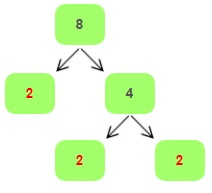## How to Find the Least Common Multiple (LCM)

Whenever we multiply two whole numbers, we get another number. Now, basically, the numbers we multiplied to get the product, are called the factors of the product. So, for example, $$2 \times 3 = 6$$, this implies that $$2$$ and $$3$$ are the factors of $$6$$. Another conclusion which we can draw from this is, that factors of a number completely divide the number without leaving any remainder.

For ex: Let’s consider the number $$24$$. Now $$24$$ can be divided into factors $$6$$ and $$4$$. Also $$6$$ can be further factorized into $$3$$ and $$2$$. Moreover, $$4$$ can also be factorized into $$2$$ and $$2$$. So, from this we can see that the other factors of $$24$$ are $$3$$, $$8$$ and $$2$$. This is because $$12 \times 2 = 8 \times 3 = 3 \times 8=24$$. Now, let’s learn some facts about factors.

• Each and every number has a smallest factor which is $$1$$.
• Every number has a minimum of two factors that is $$1$$ and the number itself.
• Now, such numbers which have only two factors, i.e., $$1$$ and the number itself are called prime numbers.

#### Prime Factorization

Prime factorization is defined as the product of all the prime factors of a number whose multiplication gives the number itself. Moreover, sometimes to write the prime factors of a number, we may have to repeat the number. So, for example, the factors of $$8$$ are $$1$$ and $$2$$, but to represent $$8$$ we use $$8=2 \times 2 \times 2$$.How to Calculate Prime Factorization of 8?

#### How to Find the Least Common Multiple (LCM)

To find the Least Common Multiple, follow these steps:

• First, find the GCF between the two numbers.
• Next, divide any of those 2 numbers by the GCF.
• Finally, multiply the answer by the other number.

For example, $$100 = 2 \times 2 \times 5 \times 5$$ and $$50 = 2 \times 5 \times 5$$. so $$GCF (100,50) = 2 \times 5 \times 5 = 50$$.
Now, $$100 \div 50 = 2$$. Therefore,$$LCM (100,50) = 50 \times 2=100$$.

### Exercises for Finding Least Common Multiple

1) $$LCM (52, \ 18) =$$

2) $$LCM (50, \ 15) =$$

3) $$LCM (81, \ 30) =$$

4) $$LCM (74, \ 58) =$$

5) $$LCM (8, \ 28) =$$

6) $$LCM (15, \ 35) =$$

7) $$LCM (37, \ 74) =$$

8) $$LCM (30, \ 72) =$$

9) $$LCM (23, \ 46) =$$

10) $$LCM (38, \ 40) =$$

1) $$LCM (52, \ 18) = \ \color{red}{ 18 \ \div \ 2 \ \times \ 52 \ = 468}$$
Solution:
Step 1: Find the GCF of the numbers. GCF: $$(52, \ 18) = \ 2$$
Step 2: Divide that GCF into either number. $$18 \div 2 = 9$$
Step 3: Take that answer and multiply it by the other number. $$9 \times 52 = 468$$
Greatest Common Factor of 52 and 18
2) $$LCM (50, \ 15) = \ \color{red}{50 \ \div \ 5 \ \times \ 15 \ = 150}$$
Solution:
Step 1: Find the GCF of the numbers. GCF : $$(50, \ 15) = \ 5$$
Step 2: Divide that GCF into either number. $$50 \div 5 = 10$$
Step 3: Take that answer and multiply it by the other number. $$10 \times 15 = 150$$
Greatest Common Factor of 50 and 15
3) $$LCM (81, \ 30) = \ \color{red}{30 \ \div \ 3 \ \times \ 81 \ = 810}$$
Solution:
Step 1: Find the GCF of the numbers. GCF : $$(81, \ 30) = \ 3$$
Step 2: Divide that GCF into either number. $$30 \div 3 = 10$$
Step 3: Take that answer and multiply it by the other number. $$10 \times 81 = 810$$
Greatest Common Factor of 81 and 30
4) $$LCM (74, \ 58) = \ \color{red}{58 \ \div \ 2 \ \times \ 74 \ = 2146}$$ 5) $$LCM (8, \ 28) = \ \color{red}{28 \ \div \ 4 \ \times \ 8 \ = 56}$$ 6) $$LCM (15, \ 35) = \ \color{red}{35 \ \div \ 5 \ \times \ 15 \ = 105}$$ 7) $$LCM (37, \ 74) = \ \color{red}{74 \ \div \ 37 \ \times \ 37 \ = 74}$$ 8) $$LCM (30, \ 72) = \ \color{red}{72 \ \div \ 6 \ \times \ 30 \ = 360}$$ 9) $$LCM (23, \ 46) = \ \color{red}{46 \ \div \ 23 \ \times \ 23 \ = 46}$$ 10) $$LCM (38, \ 40) = \ \color{red}{40 \ \div \ 2 \ \times \ 38 \ = 760}$$

## Least Common Multiple Quiz

### SSAT Upper Level Mathematics Formulas

$6.99$5.99

### TSIA2 Math Practice Workbook

$25.99$14.99

### TABE 11 & 12 Math Study Guide

$20.99$15.99

### Comprehensive Common Core Grade 8 Math Practice Book

$19.99$14.99